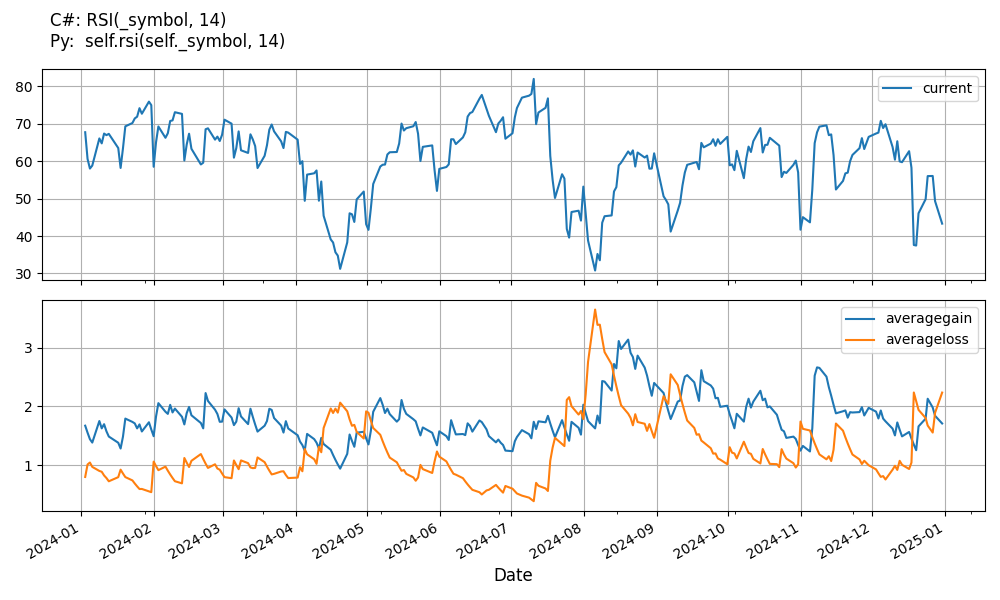# Supported Indicators

## Relative Strength Index

### Introduction

This indicator represents the Relative Strength Index (RSI) developed by K. Welles Wilder. You can optionally specified a different moving average type to be used in the computation

To view the implementation of this indicator, see the LEAN GitHub repository.

### Using RSI Indicator

To create an automatic indicators for RelativeStrengthIndex, call the RSI helper method from the QCAlgorithm class. The RSI method creates a RelativeStrengthIndex object, hooks it up for automatic updates, and returns it so you can used it in your algorithm. In most cases, you should call the helper method in the Initialize method.

public class RelativeStrengthIndexAlgorithm : QCAlgorithm
{
private Symbol _symbol;
private RelativeStrengthIndex _rsi;

public override void Initialize()
{
_rsi = RSI("SPY", 14);
}

public override void OnData(Slice data)
{
{
// The current value of _rsi is represented by itself (_rsi)
// or _rsi.Current.Value
Plot("RelativeStrengthIndex", "rsi", _rsi);
// Plot all properties of rsi
Plot("RelativeStrengthIndex", "averageloss", _rsi.AverageLoss);
Plot("RelativeStrengthIndex", "averagegain", _rsi.AverageGain);
}
}
}
class RelativeStrengthIndexAlgorithm(QCAlgorithm):
def Initialize(self) -> None:
self.rsi = self.RSI("SPY", 14)

def OnData(self, slice: Slice) -> None:
# The current value of self.rsi is represented by self.rsi.Current.Value
self.Plot("RelativeStrengthIndex", "rsi", self.rsi.Current.Value)
# Plot all attributes of self.rsi
self.Plot("RelativeStrengthIndex", "averageloss", self.rsi.AverageLoss.Current.Value)
self.Plot("RelativeStrengthIndex", "averagegain", self.rsi.AverageGain.Current.Value)


The following reference table describes the RSI method:

### RSI()1/1

            RelativeStrengthIndex QuantConnect.Algorithm.QCAlgorithm.RSI (
Symbol                           symbol,
Int32                            period,
*MovingAverageType               movingAverageType,
*Nullable<Resolution>      resolution,
*Func<IBaseData, Decimal>  selector
)


Creates a new RelativeStrengthIndex indicator. This will produce an oscillator that ranges from 0 to 100 based on the ratio of average gains to average losses over the specified period.

If you don't provide a resolution, it defaults to the security resolution. If you provide a resolution, it must be greater than or equal to the resolution of the security. For instance, if you subscribe to hourly data for a security, you should update its indicator with data that spans 1 hour or longer.

The following table describes the MovingAverageType enumeration members:

You can manually create a RelativeStrengthIndex indicator, so it doesn't automatically update. Manual indicators let you update their values with any data you choose.

Updating your indicator manually enables you to control when the indicator is updated and what data you use to update it. To manually update the indicator, call the Update method with time/number pair, or an IndicatorDataPoint. The indicator will only be ready after you prime it with enough data.

public class RelativeStrengthIndexAlgorithm : QCAlgorithm
{
private Symbol _symbol;
private RelativeStrengthIndex _rsi;

public override void Initialize()
{
_rsi = new RelativeStrengthIndex(14);
}

public override void OnData(Slice data)
{
if (data.Bars.TryGeValue(_symbol, out var bar))
{
_rsi.Update(bar.EndTime, bar.Close);
}

{
// The current value of _rsi is represented by itself (_rsi)
// or _rsi.Current.Value
Plot("RelativeStrengthIndex", "rsi", _rsi);
// Plot all properties of rsi
Plot("RelativeStrengthIndex", "averageloss", _rsi.AverageLoss);
Plot("RelativeStrengthIndex", "averagegain", _rsi.AverageGain);
}
}
}
class RelativeStrengthIndexAlgorithm(QCAlgorithm):
def Initialize(self) -> None:
self.rsi = RelativeStrengthIndex(14)

def OnData(self, slice: Slice) -> None:
bar = slice.Bars.get(self.symbol)
if bar:
self.rsi.Update(bar.EndTime, bar.Close)

# The current value of self.rsi is represented by self.rsi.Current.Value
self.Plot("RelativeStrengthIndex", "rsi", self.rsi.Current.Value)
# Plot all attributes of self.rsi
self.Plot("RelativeStrengthIndex", "averageloss", self.rsi.AverageLoss.Current.Value)
self.Plot("RelativeStrengthIndex", "averagegain", self.rsi.AverageGain.Current.Value)


To register a manual indicator for automatic updates with the security data, call the RegisterIndicator method.

public class RelativeStrengthIndexAlgorithm : QCAlgorithm
{
private Symbol _symbol;
private RelativeStrengthIndex _rsi;

public override void Initialize()
{
_rsi = new RelativeStrengthIndex(14);
RegisterIndicator(_symbol, _rsi, Resolution.Daily);
}

public override void OnData(Slice data)
{
{
// The current value of _rsi is represented by itself (_rsi)
// or _rsi.Current.Value
Plot("RelativeStrengthIndex", "rsi", _rsi);
// Plot all properties of rsi
Plot("RelativeStrengthIndex", "averageloss", _rsi.AverageLoss);
Plot("RelativeStrengthIndex", "averagegain", _rsi.AverageGain);
}
}
}
class RelativeStrengthIndexAlgorithm(QCAlgorithm):
def Initialize(self) -> None:
self.rsi = RelativeStrengthIndex(14)
self.RegisterIndicator(self.symbol, self.rsi, Resolution.Daily)

def OnData(self, slice: Slice) -> None:
# The current value of self.rsi is represented by self.rsi.Current.Value
self.Plot("RelativeStrengthIndex", "rsi", self.rsi.Current.Value)
# Plot all attributes of self.rsi
self.Plot("RelativeStrengthIndex", "averageloss", self.rsi.AverageLoss.Current.Value)
self.Plot("RelativeStrengthIndex", "averagegain", self.rsi.AverageGain.Current.Value)


The following reference table describes the RelativeStrengthIndex constructor:

### RelativeStrengthIndex()1/2

            RelativeStrengthIndex QuantConnect.Indicators.RelativeStrengthIndex (
int                 period,
*MovingAverageType  movingAverageType
)


Initializes a new instance of the RelativeStrengthIndex class with the specified name and period.

### RelativeStrengthIndex()2/2

            RelativeStrengthIndex QuantConnect.Indicators.RelativeStrengthIndex (
string              name,
int                 period,
*MovingAverageType  movingAverageType
)


Initializes a new instance of the RelativeStrengthIndex class with the specified name and period.

### Visualization

The following image shows plot values of selected properties of RelativeStrengthIndex using the plotly library.You can also see our Videos. You can also get in touch with us via Discord.